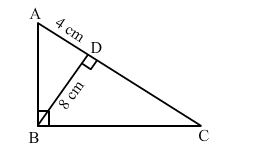# In the given figure, ∠ABC = 90° and BD ⊥ AC.

Question:

In the given figure, ∠ABC = 90° and BD ⊥ AC. If BD = 8 cm, AD = 4 cm, find CD.Solution:

It is given that ABC is a right angled triangle and BD is the altitude drawn from the right angle to the hypotenuse.

In $\triangle D B A$ and $\triangle D C B$, we have :

$\angle B D A=\angle C D B$

$\angle D B A=\angle D C B=90^{\circ}$

Therefore, by AA similarity theorem, we get:

$\triangle D B A \sim \triangle D C B$

$\Rightarrow \frac{B D}{C D}=\frac{A D}{B D}$

$\Rightarrow C D=\frac{B D^{2}}{A D}$

$C D=\frac{8 \times 8}{4}=16 \mathrm{~cm}$